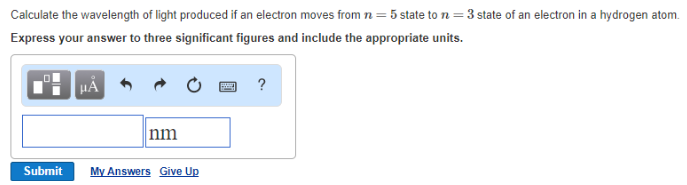# Problem: Calculate the wavelength of light produced if an electron moves from n = 5 state to n = 3 state of an electron in a hydrogen atom.Express your answer to three significant figures and include the appropriate units.

🤓 Based on our data, we think this question is relevant for Professor Drolet's class at OREGONSTATE.

###### FREE Expert Solution###### Problem Details

Calculate the wavelength of light produced if an electron moves from n = 5 state to n = 3 state of an electron in a hydrogen atom.

Express your answer to three significant figures and include the appropriate units.